Grab a pencil - it might come in handy when playing this quiz!

# Fractions (Multiplying with Mixed Numbers)

This Math quiz is called 'Fractions (Multiplying with Mixed Numbers)' and it has been written by teachers to help you if you are studying the subject at middle school. Playing educational quizzes is a fabulous way to learn if you are in the 6th, 7th or 8th grade - aged 11 to 14.

It costs only \$12.50 per month to play this quiz and over 3,500 others that help you with your school work. You can subscribe on the page at Join Us

The best part about multiplying with fractions is that you do not need to find the “common denominator”. Rather, when multiplying fractions you simply multiply the numerators and then you multiply the denominators. See the example below before tackling the following ten questions.

Go straight to Quiz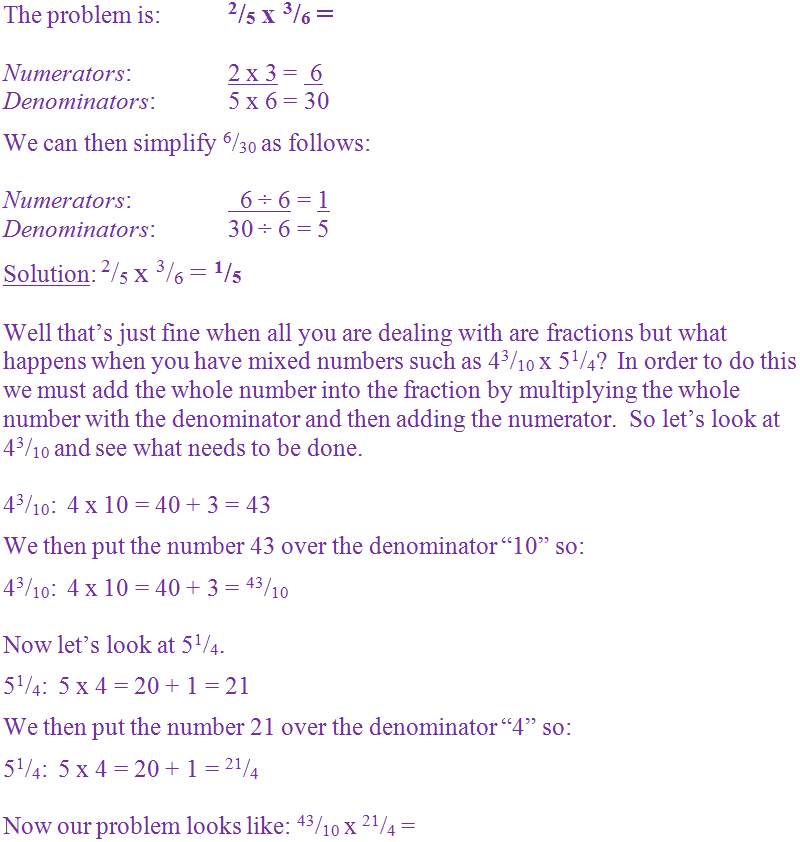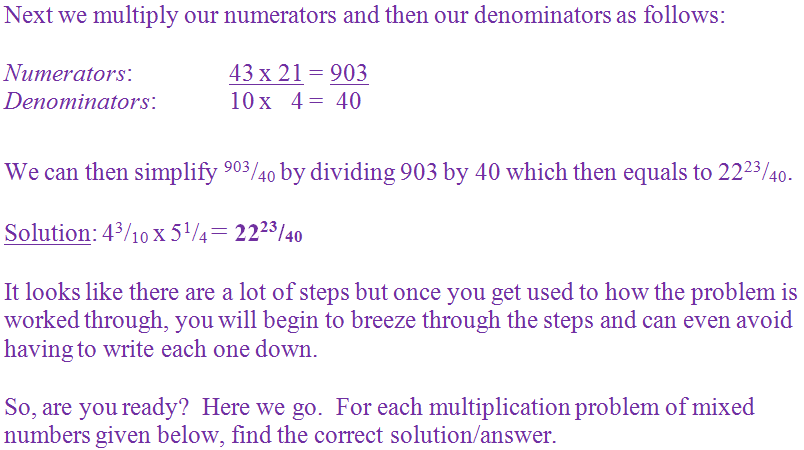1.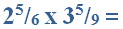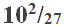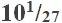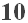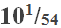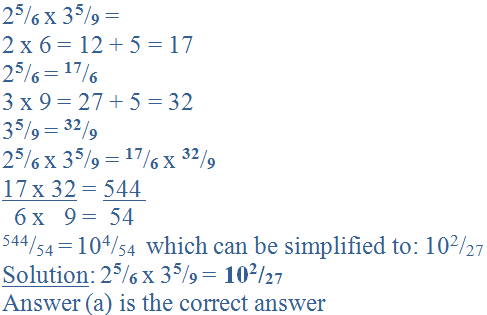2.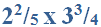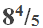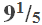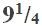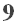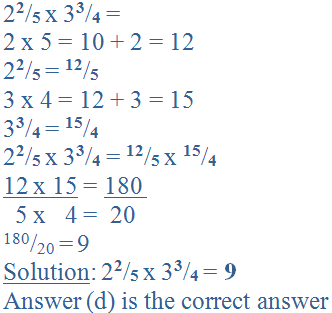3.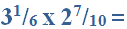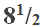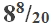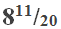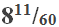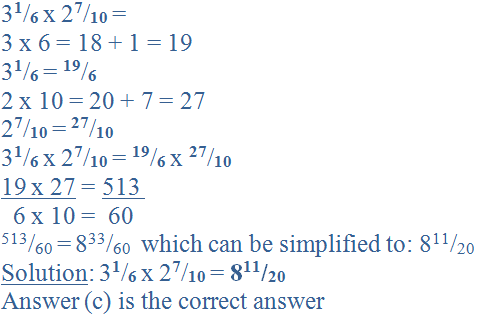4.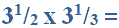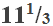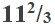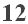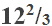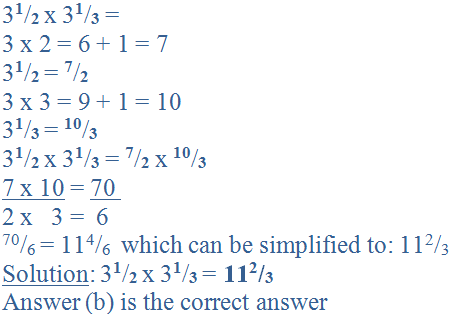5.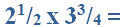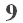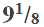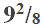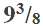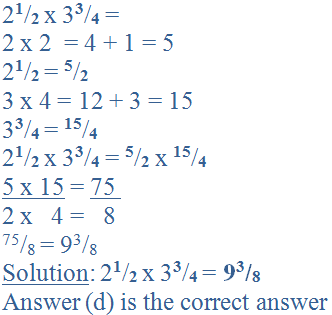6.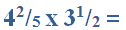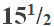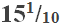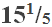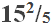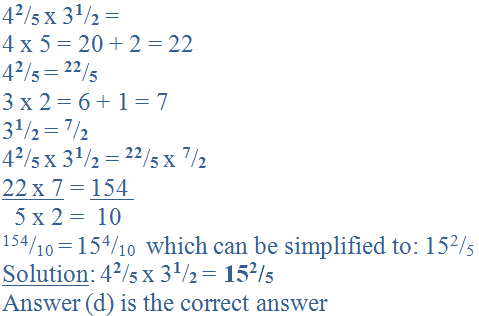7.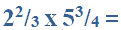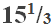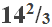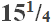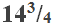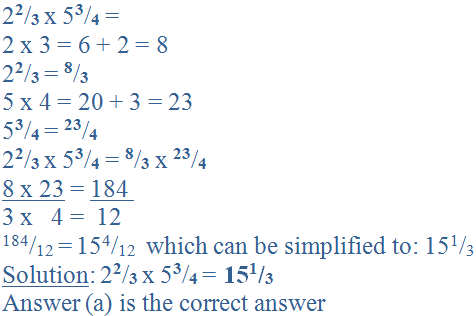8.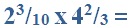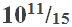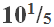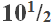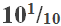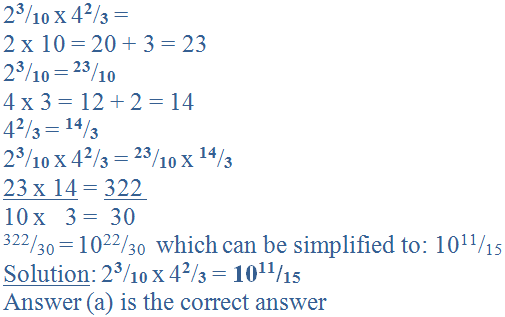9.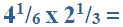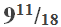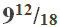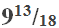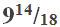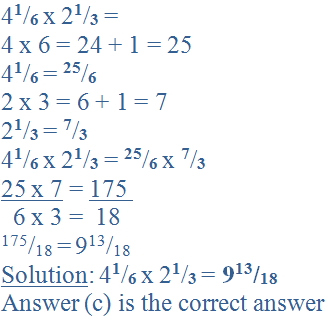10.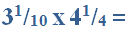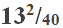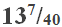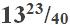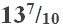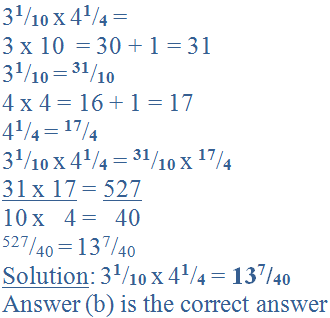Author:  Christine G. Broome